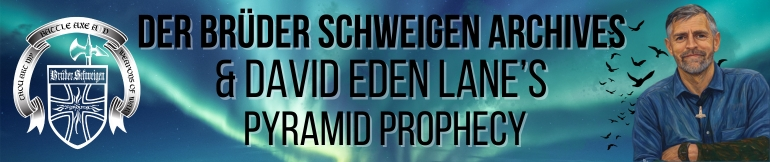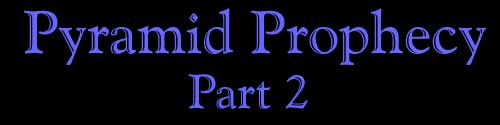The number of the Man is also found in his name as a number pyramid. The number pyramid of the word "David" runs forward and backward from a center point and demonstrates both 666 and his birth year, around the six pointed Star of David, along with 74, the first function of the 666 Sun Square.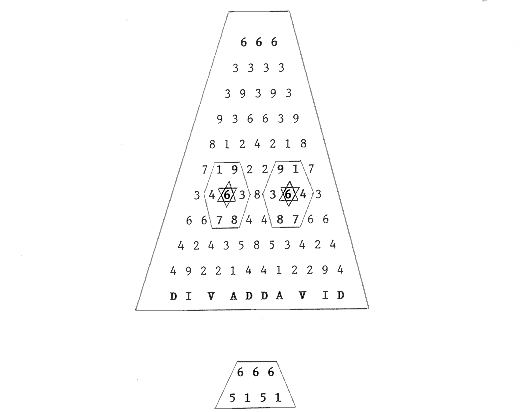Here it should be noted that the exact angle of the Great Pyramid is 51 degrees, 51 minutes from horizontal because 5151 forms this number pyramid: he equation 88² equals 7744 is concealed in the divisions of the verses in the Bible book called The Song of Solomon. The first chapter has 17 verses, the second has 17 verses, the third has 11 verses, the fourth has 16 verses, the fifth has 16 verses, the sixth has 13 verses, and the seventh has 13 verses. 17 17 11 16 16 13 13 1+7=8 1+7=8 1+1=2 1+6=7 1+6=7 1+3=4 1+3=4 8 8 ² 7 7 4 4 The name David appears once in the Song of Solomon as word number 1056 in the equation, because 88 x 12 = 1056. And because by adding 1056 to each side of the equation we get the prophecy: 882 7744 +1056 +1056 BIRTH YEAR 1938 8800 88 PRECEPTS The 7 chapters forming the equation have 2298 words. An additional chapter with 14 verses has 360 words. Because 2298 minus 360 equals 1938. The 14 represents the 14 Words. Here is the pyramid of David Lane, the full name of the Man of the prophecy, combined with his birth year of 1938: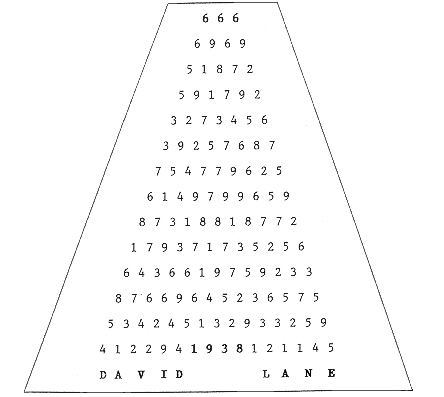In the Book of Daniel, Chapter 5, appear seven emphasized words whose true meaning is disguised with irrelevant rhetoric. The words are mene mene tekel upharsin mene tekel peres. They are designed to be written with staggered starting points like one side of a pyramid.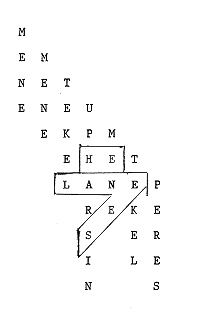Daniel is an anagram; remove the "I" and the "D", I.D., and the letters LANE remain: I. D. LANE Next we will look at the "Key of David" found in Revelation 3:7. That key is the number 749, which represents 74 x 9 = 666, the Magic Square of the Sun. First note that there are 1189 chapters in the Bible because they form a code wheel. Count all 1189 and start over. When you get to 749 it will total 1938. 749 + 1189 = 1938. The name David appears three times in Revelation. The first time in Chapter 3:7, which references the "Key of David." He also appears in Chapter 5:5 and in Chapter 22:16. There are 1666 words in the three chapters to encode 666. Count to David in each chapter and the total words are 749, because 74 x 9 = 666. Count to David in each verse. He is word 28 and 22 and 24 for a total of 74. They also form the below pyramid: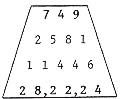The Great Pyramid is found in Isaiah 19:19, because 19 + 19 = 38. It is called an altar to the Lord in the land of Egypt. Count 38 verses from Isaiah 19:19, which corresponds with the 38° from vertical in the pyramid angle. It is Isaiah 22:9, and contains the word David the first time after the pyramid reference. Continue counting to the 51st verse from Isaiah 19:19, corresponding to the 51° from horizontal in the pyramid angle. It is Isaiah 22:22, the second time David appears. This verse references the Key of the House of David, which is the Great Pyramid, Solomon's Temple, and the Great Seal of the United States. The two verses, Isaiah 22:9 and 22:22 are a key. Count to David from the front of each verse. The total words are 19. Count to David from the end of each verse. The total words are 38. Time after time we find 19 tied to 38, and 38 tied to 51, as 38 x 51 = 1938. Thus we find 19 verses in the 38th Psalm of David, which is Psalm 51. Or, the 38th David in the K.J.V. is in I Samuel 17:50, while the 51st David in the K.J.V. is in I Samuel 18:8, because 1750 + 188 = 1938! David Eden Lane